$\stackrel{\mathrm{H}}{\stackrel{|}{{\mathrm{CH}}_{2}}}-\stackrel{\mathrm{Cl}}{\stackrel{|}{{\mathrm{CH}}_{2}}}\underset{\mathrm{alk}.}{\overset{\mathrm{NaOH}}{\to }}{\mathrm{CH}}_{2}={\mathrm{CH}}_{2}$

Most probable mechanism for this reaction is-

1. E1

2. E2

3. $E{1}_{CB}$

4. $\alpha$ elimination

Concept Questions :-

Alkanes, Alkenes and Alkynes - Chemical Properties
High Yielding Test Series + Question Bank - NEET 2020

Difficulty Level:

Which will undergo reaction with ammonical $AgN{O}_{{3}^{-}}$

(A)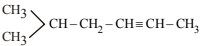(B)

(C)

(D)

Concept Questions :-

Alkanes, Alkenes and Alkynes - Chemical Properties
High Yielding Test Series + Question Bank - NEET 2020

Difficulty Level:

The no. of isomeric sodium salt that will be required to obtain neopentane.

(A) 3                                                   (B) 1

(C) 4                                                   (D) 6

High Yielding Test Series + Question Bank - NEET 2020

Difficulty Level:

Propadiene, ${C}_{3}{H}_{4}$ molecule contains –

(A) Two $s{p}^{2}$and one sp hybrid carbon

(B) One $s{p}^{2}$ and two sp hybrid carbons

(C) One $s{p}^{2}$ and three sp hybrid carbons

(D) None of these

Concept Questions :-

Aliphatic Hydrocarbon- physical properties
High Yielding Test Series + Question Bank - NEET 2020

Difficulty Level:

In Wurtz reaction if we take  then product, will be-

(A) Propane + Ethane                                                  (B) Propane

(C) Propane + Ethane + Butane                                   (D) Propane + Butane

Concept Questions :-

Alkanes, Alkenes and Alkynes -Methods of Preparation
High Yielding Test Series + Question Bank - NEET 2020

Difficulty Level:

The compound most likely to decolourise a solution of
alkaline $KMn{O}_{4}$ is –

(A)                                                (B)  ${C}_{10}{H}_{8}$

(C) $\left(C{H}_{3}{\right)}_{4}C$                                                    (D)

Concept Questions :-

Alkanes, Alkenes and Alkynes - Chemical Properties
High Yielding Test Series + Question Bank - NEET 2020

Difficulty Level:

Sabatier Senderen’s reaction is an example of-

(A) Non thermic reaction                                 (B) Exothermic reaction

(C) Endothermic reaction                                 (D) None

Concept Questions :-

Alkanes, Alkenes and Alkynes - Chemical Properties
High Yielding Test Series + Question Bank - NEET 2020

Difficulty Level:

The type of reaction and intermediate of decarboxylation reaction are.-

(A)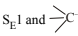(B)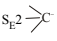(C)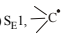(D)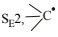Concept Questions :-

Alkanes, Alkenes and Alkynes - Chemical Properties
High Yielding Test Series + Question Bank - NEET 2020

Difficulty Level:

Which of the following has maximum melting point-

(A)                                   (B) ${C}_{5}{H}_{12}$
(C)                                   (D) ${C}_{7}{H}_{16}$

Concept Questions :-

Alkanes, Alkenes and Alkynes - Chemical Properties
High Yielding Test Series + Question Bank - NEET 2020

Difficulty Level:

Reaction of alkene and peracid gives oxyrane. This reaction is named as-

1. Peroxidation                                    2. Oxidation

3. epoxydation                                    4. None